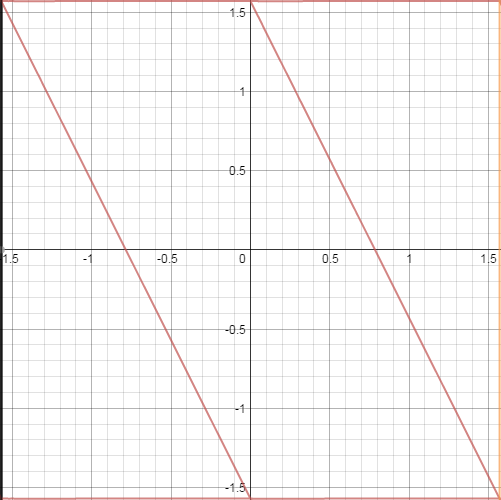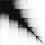# Sinx... with a twist!

I'm sure almost all of you have seen this well-known identity:

$\displaystyle \sin ^{ 2 }{ x } +\cos ^{ 2 }{ x } =1$

But what if this is made into something you can graph? Consider

$\displaystyle \sin ^{ 2 }{ x } +\sin ^{ 2 }{ (x+y) } =1$

In this case, we are looking for $y$ values such that $\left| \cos{ x } \right| =\left| \sin{ (x+y) } \right|$. For now, lets say that $(x,y)\in [ -\frac { \pi }{ 2 } , \frac { \pi }{ 2 } ]$.

The solutions we have can be found from:

$\displaystyle \left| \cos { \left( \pm \frac {\pi}{2} \pm x \right)} \right|= \left| \sin {x} \right|$

This gives $y=\{\pm \frac{\pi}{2}, \pm \frac{\pi}{2}-2x\}$. Here's what that looks like:Since $\sin^{2}{x}$ is periodic (that is, we can add $\pi$ to x and get the same results for $y$), the graph is this parallelogram tessellated on the plane! Cool, huh?

But wait... what if we didn't square the terms? It gets harder then...

$\displaystyle \sin{x}+\sin{\left(x+y\right)}=1$

Intuitively, we're going to get some shape that is tiled on the plane but half as densely - due to the period being twice as long.

I'll update this note with the entire process later, but essentially the graph of this can be found by the union of $\displaystyle y=2\pi k-\arcsin{\left( 1-\sin{x}\right)}-x+\pi$ $\displaystyle y=\arcsin{\left( 1-\sin{x}\right)}-x-2\pi k$ for $k \in Z$. This results in a shape somewhat like a bean.Note by Dylan Pentland
6 years, 2 months ago

This discussion board is a place to discuss our Daily Challenges and the math and science related to those challenges. Explanations are more than just a solution — they should explain the steps and thinking strategies that you used to obtain the solution. Comments should further the discussion of math and science.

When posting on Brilliant:

• Use the emojis to react to an explanation, whether you're congratulating a job well done , or just really confused .
• Ask specific questions about the challenge or the steps in somebody's explanation. Well-posed questions can add a lot to the discussion, but posting "I don't understand!" doesn't help anyone.
• Try to contribute something new to the discussion, whether it is an extension, generalization or other idea related to the challenge.

MarkdownAppears as
*italics* or _italics_ italics
**bold** or __bold__ bold
- bulleted- list
• bulleted
• list
1. numbered2. list
1. numbered
2. list
Note: you must add a full line of space before and after lists for them to show up correctly
paragraph 1paragraph 2

paragraph 1

paragraph 2

[example link](https://brilliant.org)example link
> This is a quote
This is a quote
    # I indented these lines
# 4 spaces, and now they show
# up as a code block.

print "hello world"
# I indented these lines
# 4 spaces, and now they show
# up as a code block.

print "hello world"
MathAppears as
Remember to wrap math in $$ ... $$ or $ ... $ to ensure proper formatting.
2 \times 3 $2 \times 3$
2^{34} $2^{34}$
a_{i-1} $a_{i-1}$
\frac{2}{3} $\frac{2}{3}$
\sqrt{2} $\sqrt{2}$
\sum_{i=1}^3 $\sum_{i=1}^3$
\sin \theta $\sin \theta$
\boxed{123} $\boxed{123}$

Sort by:

Again, some interesting work!

Good luck investigating $\sin{x}+\sin(x+y)=1.$ If I had to study that curve, I would look at $\sin{x}+\sin{y}=1$ first... you can always "shear" your result to get the original curve. Also, it may help to study $\sin{x}+\sin{y}=b$ for various values of $b$ between $-2$ and $2$, including $b=0$... that way you get a feel for the problem.

Keep us posted!

- 6 years, 2 months ago

Respected Sir, please do accept my regards. I am a brilliant frequent and find most of your problems very challenging and interesting. I am sure that I can never reach your level in life,still, I do ask," What makes you so strong in Mathematics?" Do please share your strategies,if any, like the resources you use, and the very others.

Thanks!@Otto Bretscher

- 6 years, 2 months ago

Thank you, Swapnil, for your kind words. You know, being "strong" in math is a relative thing... I have been in many advanced classes and seminars in Zurich and Cambridge, MA, where I felt like (and was) the dumbest guy in the room. When I was about 30, I happened to take an algebra class at Harvard (with just a few students) where one of my class mates was Noam Elkies, then about 20 years old, a great math genius...I felt like a total idiot in his presence.

It's not about comparing yourself to others, but enjoying your studies and doing meaningful work (whether it's learning, teaching, or doing research). There is a big portion of luck involved in becoming a really great mathematician (I'm surrounded by them here in Cambridge, MA): You have to be at the right place at the right time (Goettingen before WWII, Paris in the 60s, Moscow in the 80s), finding the right teachers, focus on the right questions at a very early age. Every honest biography of a great mathematician contains a sentence like "I have been incredibly lucky with my teachers". But even those of us who don't make it to that level (almost all of us) can have a fun-filled and meaningful professional life... in my case I have dedicated myself mostly to teaching.

In my opinion, Brilliant is actually a great way to "think about math"... I always tell my students to "do the problems they can't do". If you know in advance which technique to use, a problem is no longer interesting. Try the most challenging problems...

I also tell my ambitious students to read biographies of great mathematicians. Right now I read Edward Frenkel's "Love & Math", and I'm very impressed (I taught with Prof. Frenkel for a few years at Harvard, mostly Linear Algebra)... maybe you can get a copy!

Good luck, take care, and stay in touch!

- 6 years, 1 month ago

Good note! Keep posting!

- 6 years, 2 months ago